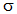# Mean Stress Corrections in FatigueFebruary 12, 2016 By: Michael Bak

A number of our blogs have discussed different topics related to fatigue, including accounting for fatigue data scatter, calculating fatigue life when the load history is complex, predicting fatigue life of welded structures, and calculating fatigue in a random vibration environment.  Another important topic in fatigue is accounting for the effect of non-zero mean stress, which will be discussed here.

Mean stress is equal to the average of the maximum and minimum stress during a fatigue load cycle.  Much of fatigue data is generated assuming a zero mean stress, which means that the load cycle is completely reversed.  The stress ratio, also referred to as R or R-ratio, is defined as the minimum stress over the maximum stress and is used to quantify the mean stress.  A stress ratio of -1 represents a fully reversed loading.  An example representation of zero mean stress is shown in Figure 1 above.

Conditions of fully reversed loading are rarely met in engineering practice. For more realistic and complex loading, the mean stress will not be zero. How should the mean stress effect be included in a fatigue life calculation? There are three main approaches:

1. Obtain S-N data for multiple stress ratios.
2. Plot alternating stress versus mean stress on a Goodman Diagram.
3. Use the Goodman approach to calculate an effective alternating stress.

The first approach is the best one if available: obtain the fatigue S-N data for a range of stress ratios, and then use the appropriate curve, or interpolate between curves, to obtain cycles to failure. Fatigue analysis codes like nCode DesignLife can accept multiple stress ratio curves, and then apply that data to the alternating stress and mean stress at every point in a finite element model to calculate fatigue life. The drawback is that this data often does not exist. An example of S-N data for various stress ratios is shown in Figure 2.

Request a free FEA Consulting Consultation today!

Figure 2: Wrought Aluminum Alloy 7075-T6510 R-Ratio S-N Data from nCode

## meanstress2.pngThe second approach is to create a Goodman Diagram that defines a failure surface for varying mean stress.  A Goodman Diagram is typically constructed by plotting mean stress on the X-axis and alternating stress on the Y-axis.  The first point on the line is plotted at a mean stress (m) of zero and an alternating stress (a) equal to the endurance limit (e).  The second point is an alternating stress of zero and a mean stress equal to the ultimate tensile stress (u). Connecting the points with a straight line defines the Goodman line, with the graphical representation and equation as shown in Figure 3.  Any point that falls below the Goodman line will not fail in fatigue; any point that falls outside the Goodman line will eventually fail in fatigue.

Figure 3: Goodman Diagram

## meanstress3.pngNote that this approach does not predict cycles to failure; however, it will show if there is a potential for fatigue failure.  For example, a finite element analysis can be performed by applying the minimum and maximum load conditions in the cycle.  The mean and alternating stresses can be found at every point in the model and plotted on the Goodman Diagram, such as the example in Figure 4.  In addition to determining if the design is susceptible to fatigue failure, the critical locations can be obtained.

Figure 4: Finite Element Results Plotted on Goodman Diagram

## meanstress4.pngRequest a free FEA Consulting Consultation today!

The third approach uses a Goodman approach to calculate a mean stress correction to obtain an effective alternating stress that can be used with an S-N curve of stress ratio of -1.  Knowing the alternating stress, the mean stress, and the ultimate tensile stress, the following Goodman equation and accompanying graph in Figure 5 is used to find the effective alternating stress (eff) for a stress ratio of -1:

Figure 5: Goodman Mean Stress Correction

## meanstress5.pngThis correction method can be used to include the mean stress effect in fatigue codes like nCode DesignLife when the S-N data is only available for fully reversed loading.  Note that without this correction, fatigue life prediction would use the alternating stress magnitude ofa, noteff.

The Goodman line is generally not considered accurate for all cases, and variations on Goodman have been proposed, including the parabolic Gerber line, and the Soderberg line which uses the yield stress instead of the ultimate stress to define the second point on the curve.  In addition, modifications to these lines, particularly changing the behavior in the compressive mean stress region, are available.  But all of these variations can be used in the same manner as described in this blog.

Finally, as was stated in a previous blog on fatigue of welds, mean stress effects are usually not considered significant for welded regions since high residual stresses in the weld wash out the mean stress effect.

The method to choose for a particular application will vary based on several factors:

• The availability and features of a fatigue analysis code.
• Existence of S-N data that varies with R-ratio.
• The accuracy of the stress calculation.
• The time and funding available to assess fatigue life.

Request a free FEA Consulting Consultation today!

In my career, I have used all three procedures in various FEA consulting projects, including using multiple R-ratio S-N data for critical aerospace components, the Goodman Diagram to assess medical stent designs, and mean stress corrections for fatigue life of mortar tubes.